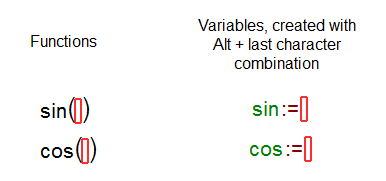# Variables

Variable is a mathematical expression symbol that represents quantity. You can define variables in MatDeck by placing := after the variable name.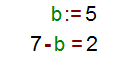As you can see, variables are displayed in green in MatDeck. They are case sensitive, which means that b and B are considered as two different variables.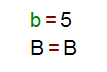When you define a variable in a document, it will be visible in the rest of that document from the row where it was placed. But if you try to use variable before it is defined, it will be considered as a symbol.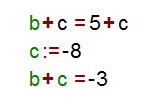You can place all types of data in variables: numerical and symbolic values, vectors, matrix, functions and units.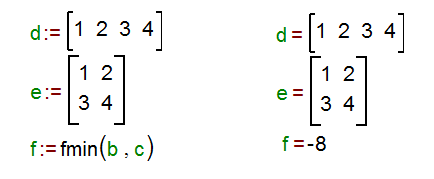When you define variables, there are some restrictions that you have to consider. Fractions, expressions, brackets and they content cannot be defined as variables.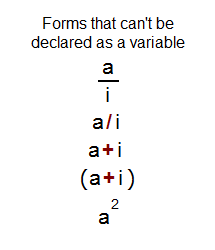If you want to define a variable with the name sin (same as a name of function) follow the following procedure. When you type the last character from the function’s name, n in this case, use Alt + last character in combination.